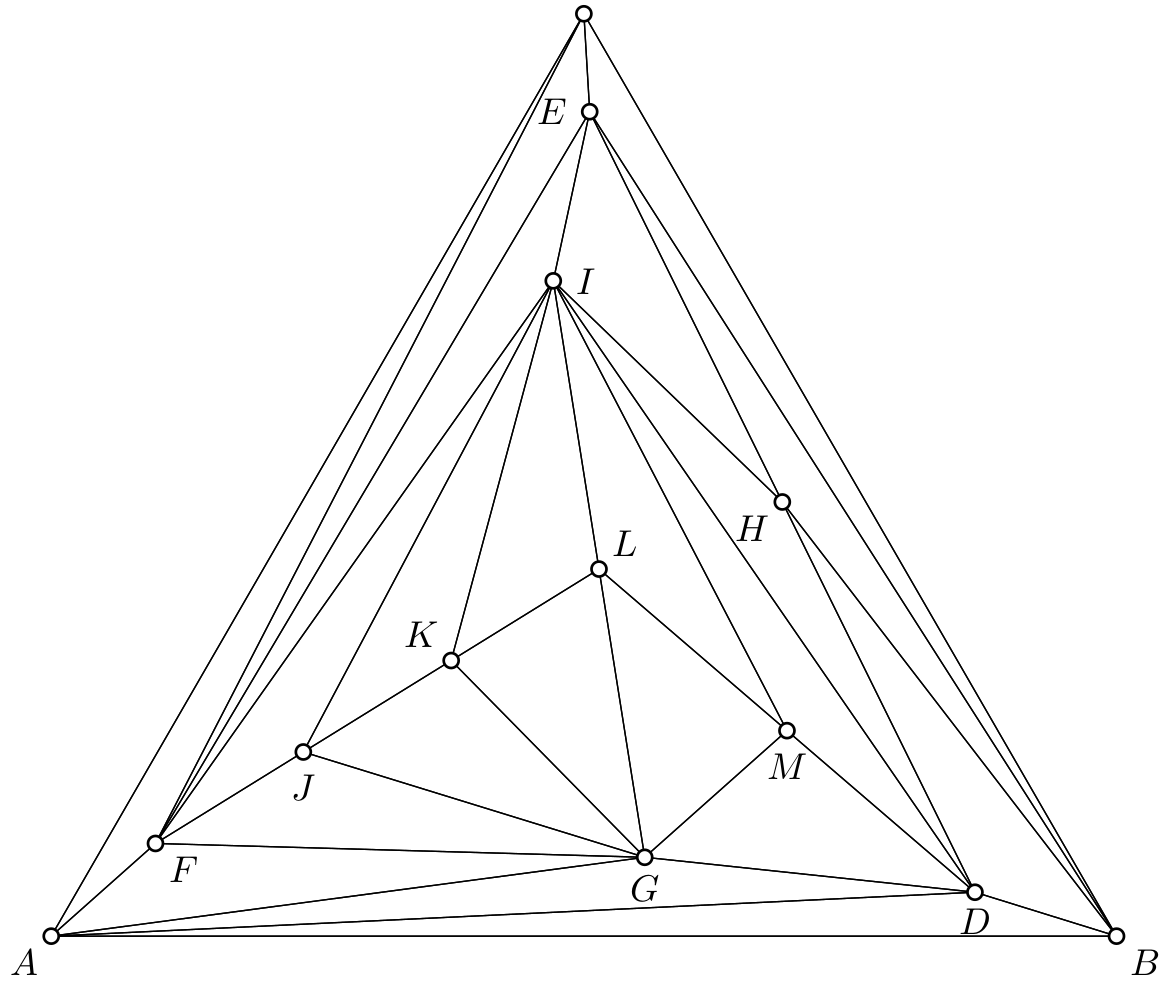# Equiareal triangulations of the triangle## 4-connected triangulations

Here is a list of equiareal embeddings combinatorial types of 4-connected triangulations of the triangle with n vertices.
Exact solutions, given as algebraic numbers.
 n=6 n=7 n=8 n=9 n=10 n=11 n=12 # of triangulations 1 1 3 13 47 217 1041

Numerical results.
 n=13 n=14 n=15 # of triangulations 5288 27844 150608